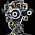7/28/2014

(OpenCV Study) OpticalFlow Gpu feature extraction and matching (GoodFeaturesToTrackDetector_GPU, gpu:: PyrLKOpticalFlow example source code)

This is example source code of Gpu mode optical flow and matching.
Source code is little bit complex in matching refine part.

function of BruteForceMatcher_GPU is added for more accurate matching.
The result is like that.

The source code is here..

...
Mat Ma = Mat::eye(3, 3, CV_64FC1);
cout << Ma << endl;
double dm = { { 1, 2, 3 }, { 4, 5, 6 }, { 7, 8, 9 } };
Mat Mb = Mat(3, 3, CV_64F, dm);
cout << Mb << endl;

//Matrix - matrix operations :
Mat Mc;
cv::add(Ma, Mb, Mc); // Ma+Mb   -> Mc
cout << Ma+Mb << endl;
cout << Mc << endl;
cv::subtract(Ma, Mb, Mc);      // Ma-Mb   -> Mc
cout << Ma - Mb << endl;
cout << Mc << endl;
Mc = Ma*Mb; //Ma*Mb;
cout << Mc << endl;

//Elementwise matrix operations :
cv::multiply(Ma, Mb, Mc);   // Ma.*Mb   -> Mc
cout << Mc << endl;
Mc = Ma.mul(Mb);
cout << Mc << endl;
cv::divide(Ma, Mb, Mc);      // Ma./Mb  -> Mc
cout << Mc << endl;
Mc = Ma + 10; //Ma + 10 = Mc
cout << Mc << endl;

//Vector products :
double va[] = { 1, 2, 3 };
double vb[] = { 0, 0, 1 };
double vc;

Mat Va(3, 1, CV_64FC1, va);
Mat Vb(3, 1, CV_64FC1, vb);
Mat Vc(3, 1, CV_64FC1, vc);

double res = Va.dot(Vb); // dot product:   Va . Vb -> res
Vc = Va.cross(Vb);    // cross product: Va x Vb -> Vc
cout << res << " " << Vc << endl;

//Single matrix operations :
Mc = Mb.t();      // transpose(Ma) -> Mb (cannot transpose onto self)
cout << Mc << endl;
cv::Scalar t = trace(Ma); // trace(Ma) -> t.val
cout << t.val << endl;
double d = determinant(Ma); // det(Ma) -> d
cout << d << endl;
Mc = Ma.inv();         // inv(Mb) -> Mc
invert(Ma, Mc);
cout << Mc << endl;

//Inhomogeneous linear system solver :
double dm2 = { { 1, 2, 3 }, { 4, 5, 6 }, { 7, 8, 9 } };
Mat A(3, 3, CV_64FC1, dm2);
Mat x(3, 1, CV_64FC1);
double vvb[] = { 14, 32, 52 };
Mat b(3, 1, CV_64FC1, vvb);
cv::solve(A, b, x, DECOMP_SVD); //// solve (Ax=b) for x
cout << x << endl;

//Eigen analysis(of a symmetric matrix) :
float f11[] = { 1, 0.446, -0.56, 0.446, 1, -0.239, -0.56, 0.239, 1 };
Mat data(3, 3, CV_32F, f11);
Mat value, vector;
eigen(data, value, vector);
cout << "Eigenvalues" << value << endl;
cout << "Eigenvectors" << endl;
cout << vector << endl;

//Singular value decomposition :
Mat w, u, v;
SVDecomp(data, w, u, v); // A = U W V^T
//The flags cause U and V to be returned transposed(does not work well without the transpose flags).
cout << w << endl;
cout << u << endl;
cout << v << endl;
...

refer to this posting
optical flow and matching using gpu
http://feelmare.blogspot.kr/2014/07/opencv-study-opticalflow-gpu-feature.html
orb feature and matching using gpu
http://feelmare.blogspot.kr/2014/07/opencv-study-orb-gpu-feature-extraction.html
surf feature and matching using gpu
http://feelmare.blogspot.kr/2014/07/opencv-study-surf-gpu-and-matching.html

1.1.